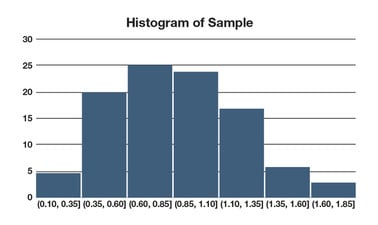# The Georgia Institute of Technology: Probability and Statistics IV: Confidence Intervals and Hypothesis Tests

This course covers two important methodologies in statistics – confidence intervals and hypothesis testing. Confidence intervals allow us to make probabilistic statements such as: “We are 95% sure that Candidate Smith’s popularity is 52% +/- 3%.” Hypothesis testing allows us to pose hypotheses and test their validity in a statistically rigorous way. For instance, “Does a new drug result in a higher cure rate than the old drug?"4 weeks
6–10 hours per week
Self-paced
Free

This course covers two important methodologies in statistics – confidence intervals and hypothesis testing.

Confidence intervals are encountered in everyday life, and allow us to make probabilistic statements such as: “Based on the sample of observations we conducted, we are 95% sure that the unknown mean lies between A and B,” and “We are 95% sure that Candidate Smith’s popularity is 52% +/- 3%.” We begin the course by discussing what a confidence interval is and how it is used. We then formulate and interpret confidence intervals for a variety of probability distributions and their parameters.

Hypothesis testing allows us to pose hypotheses and test their validity in a statistically rigorous way. For instance, “Does a new drug result in a higher cure rate than the old drug?” or “Is the mean tensile strength of item A greater than that of item B?” The second half the course begins by motivating hypothesis tests and how they are used. We then discuss the types of errors that can occur with hypothesis testing, and how to design tests to mitigate those errors. Finally, we formulate and interpret hypothesis tests for a variety of probability distributions and their parameters.

### At a glance

• Institution: GTx
• Subject: Data Analysis & Statistics
• Level: Intermediate
• Prerequisites:

Learners will be expected to come in knowing some set theory and basic calculus, as well as the material from the previous courses in this series ( A Gentle Introduction to Probability , Random Variables, and A Gentle Introduction to Statistics ). The prerequisite material is all available for you to access; and in any event, we will make the current course as self-contained as possible. In addition, this course will involve a bit of computer programming, so it would be nice to have at least a little experience in something like Excel and/or the R freeware statistical package.

• Language: English
• Video Transcript: English
• Associated programs:
• Associated skills: Statistics, Probability And Statistics, Confidence Intervals, Probability Distribution, Statistical Hypothesis Testing

# What you'll learn

Skip What you'll learn

Upon completion of this course, learners will be able to:

• Identify what a confidence interval is and how it is used
• Formulate and interpret confidence intervals for a variety of probability distributions and their parameters
• Determine what a hypothesis test is and how it is used
• Identify the types of errors that can occur with hypothesis testing, and how to design tests to mitigate those errors
• Formulate and interpret hypothesis tests for a variety of probability distributions and their parameters

# Syllabus

Skip Syllabus

“FCPS” refers to the free text, A First Course in Probability and Statistics: free access is provided via a PDF file or as a book

Module 1: Confidence Intervals
• Lesson 1: Introduction to Confidence Intervals (FCPS §6.1)
• Lesson 2: Normal Mean (variance known) (FCPS §6.2)
• Lesson 3: Difference of Two Normal Means (variances known) (FCPS §6.3)
• Lesson 4: Normal Mean (variance unknown) (FCPS §6.4)
• Lesson 5: Difference of Two Normal Means (unknown equal variances) (FCPS §6.5.1)
• Lesson 6: Difference of Two Normal Means (variances unknown) (FCPS §6.5.2)
• Lesson 7: Difference of Paired Normal Means (variances unknown) (FCPS §6.5.3)
• Lesson 8: Normal Variance (FCPS §6.6)
• Lesson 9: Ratio of Variances of Two Normals (FCPS §6.7)
• Lesson 10: Bernoulli Proportion (FCPS §6.8)
Module 2: Hypothesis Testing
• Lesson 1: Introduction to Hypothesis Testing (FCPS §7.1)
• Lesson 2: The Errors of Our Ways (FCPS §7.1)
• Lesson 3: Normal Mean Test with Known Variance (FCPS §7.2.1)
• Lesson 4: Normal Mean Test with Known Variance: Design (FCPS §7.2.2)
• Lesson 5: Two-Sample Normal Means Test with Known Variances (FCPS §7.2.3)
• Lesson 6: Normal Mean Test with Unknown Variance (FCPS §7.3.1)
• Lesson 7: Two-Sample Normal Means Tests with Unknown Variances (FCPS §7.3.2)
• Lesson 8: Two-Sample Normal Means Test with Paired Observations (FCPS §7.3.2)
• Lesson 9: Normal Variance Test (FCPS §7.4.1)
• Lesson 10: Two-Sample Normal Variances Test (FCPS §7.4.2)
• Lesson 11: Bernoulli Proportion Test (FCPS §7.4.3)
• Lesson 12: Two-Sample Bernoulli Proportions Test (FCPS §7.4.4)
• Lesson 13: Goodness-of-Fit Tests: Introduction (FCPS §7.5.1)
• Lesson 14: Goodness-of-Fit Tests: Examples (FCPS §7.5.2)
• Lesson 15 [OPTIONAL]: Goodness-of-Fit Tests: Honors Example (FCPS §7.5.3)

*What if I don’t remember much basic probability and statistics?

Answer: You can take the companion courses, “A Gentle Introduction to Probability”, “Random Variables – Great Expectations to Bell Curves”, and “A Gentle Introduction to Statistics”, which will get you going at light speed!

*Will there be much programming?

Answer: A little, but you’ll be able to handle that in Excel or Matlab or whatever your favorite programming application is. We also go over a couple of R demos, but those will be self-contained.

*What is the largest prime number to ever appear in the tile of a Top-40 song?